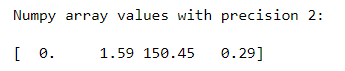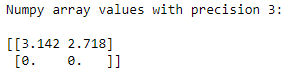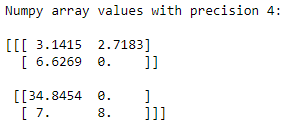# How to suppress the use of scientific notations for small numbers using NumPy?

• Last Updated : 24 Oct, 2020

Sometimes we have elements that are in scientific notations, and we have to suppress the scientific notation for simplicity. For this purpose, we call a function named as numpy.set_printoptions(). This function will help to suppress the scientific notation and display number to a certain precision.

Syntax: numpy.set_printoptions(precision=None, threshold=None, edgeitems=None, linewidth=None, suppress=None, nanstr=None, infstr=None, formatter=None, sign=None, floatmode=None, *, legacy=None)

Attention geek! Strengthen your foundations with the Python Programming Foundation Course and learn the basics.

To begin with, your interview preparations Enhance your Data Structures concepts with the Python DS Course. And to begin with your Machine Learning Journey, join the Machine Learning - Basic Level Course

Parameters:

• precision: Number of digits of precision for floating point output (default 8)
• suppress: If True, always print floating point numbers using fixed point notation, if False, then scientific notation is used when absolute value of the smallest number is < 1e-4

Rest of the parameters are optional.

Example 1:

## Python3

 `# Importing Numpy library ``import` `numpy as np`` ` `# Creating a 1-D Numpy array``num ``=` `np.array([``1.8e``-``10``, ``1.586``, ``150.45``, ``0.2855``]) `` ` `# Suppressing 1-D numpy array with precision 2 ``# using numpy.set_printoptions()``print``(``"Numpy array values with precision 2:\n"``)``np.set_printoptions(precision ``=` `2``, suppress ``=` `True``)``print``(num)`

Output:In the above example, we suppress the scientific notations for the elements of 1-D NumPy array with precision 2.

Example 2:

## Python3

 `# Importing Numpy library ``import` `numpy as np`` ` `# Creating a 2-D Numpy array``num ``=` `np.array([[``3.1415``, ``2.7182``],``                 ``[``6.6260e``-``34``, ``6.6743e``-``11``]]) `` ` `# Suppressing 2-D numpy array with precision 3 ``# using numpy.set_printoptions()``print``(``"Numpy array values with precision 3:\n"``)``np.set_printoptions(precision ``=` `3``, suppress ``=` `True``)``print``(num)`

Output:In the above example, we suppress the scientific notations for the elements of 2-D NumPy array with precision 3.

Example 3:

## Python3

 `# Importing Numpy library ``import` `numpy as np`` ` `# Creating a 3-D Numpy array``num ``=` `np.array([[[``3.141527``, ``2.718283``],``                 ``[``6.6268574``, ``6.6743e``-``11``]],``                ``[[``34.8454``, ``8.6260e``-``34``],``                 ``[``7``, ``8``]]]) `` ` `# Suppressing 3-D numpy array with precision 4``# using numpy.set_printoptions()``print``(``"Numpy array values with precision 4:\n"``)``np.set_printoptions(precision ``=` `4``, suppress ``=` `True``)``print``(num)`

Output:In the above example, we suppress the scientific notations for the elements of 3-D NumPy array with precision 4.

My Personal Notes arrow_drop_up